Sunday 15th December 2019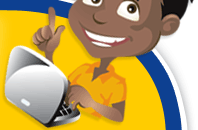# Classroom Key Stage 2 / KS2 - Maths Activities & Resources

## Welcome to the Maths section of the Key Stage 2 classroom. Within KS2 Maths you will find online @school assessment tests that will help children to enhance their learning and revise the subject of Maths at Key Stage 2 level.

•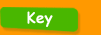•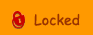•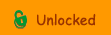••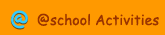•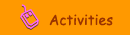•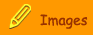###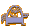Numbers Patterns & Sequences

4-digit Sequencer - Whiteboard activity for sequencing numbers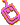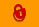Calculators and Square Numbers - Interactive activity to find square numbersDecimal Sequences - Interactive activity to sequence decimal numbersFactors - Interactive activity to find factorsFunbrain Line Jumper - Interactive activity using number lines.Funbrain Number cracker - Interactive activity to find the missing number in the sequence.Hundred Square - 10 Times Table - Interactive activity where you have to select the numbers for the 10 Times Table and spot the pattern.Hundred Square - 11 Times Table - Interactive activity where you have to select the numbers for the 11 Times Table and spot the pattern.Hundred Square - 12 Times Table - Interactive activity where you have to select the numbers for the 12 Times Table and spot the pattern.Hundred Square - 2 Times Table - Interactive activity where you have to select the numbers for the 2 Times Table and spot the pattern.Hundred Square - 3 Times Table - Interactive activity where you have to select the numbers for the 3 Times Table and spot the pattern.Hundred Square - 4 Times Table - Interactive activity where you have to select the numbers for the 4 Times Table and spot the pattern.Hundred Square - 5 Times Table - Interactive activity where you have to select the numbers for the 5 Times Table and spot the pattern.Hundred Square - 6 Times Table - Interactive activity where you have to select the numbers for the 6 Times Table and spot the pattern.Hundred Square - 7 Times Table - Interactive activity where you have to select the numbers for the 7 Times Table and spot the pattern.Hundred Square - 8 Times Table - Interactive activity where you have to select the numbers for the 8 Times Table and spot the pattern.Hundred Square - 9 Times Table - Interactive activity where you have to select the numbers for the 9 Times Table and spot the pattern.Make Your Own Number Line - Printable sheet for a number line worksheetMultiples - Interactive activity to find the multiplesMultiples 2 - Interactive activity to find the multiplesMultiples and Divisibility Rules - Interactive activity about multiples and divisibility rules.Multiples of 6, 7, 8, 9 & 11 - Interactive activity to find the multiplesNegative Sequences - Interactive activity to sequence negative numbersNumber Balance - Whiteboard activity for investigating number propertiesNumber Grid - Clipart of a number grid.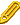Number Sequences - Year 3 - Interactive or printable activity to complete and describe number sequences.Odd and Even Numbers - Interactive activities to select the even numbersOdd and Even Rules - Work out simple additions to discover rules for adding odd and even numbers.Odd or Even - Whiteboard activity for odd and even numbersPatterns - Interactive activity to use multiples in patternsPrime Numbers - Interactive activity where you have to spot the prime numbers.Scribble Square - Interactive number grid where you can look at patterns etc.Sequences -Year 3 - Sequence the numbers displayed with this interactive activity.Smallest Common Multiple - Interactive activity to find the lowest common multipleYear 6 Sequences - Interactive activity to complete the sequences